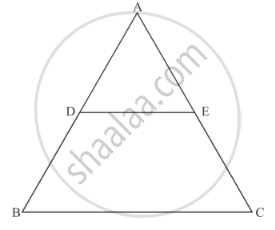Share
Notifications

View all notifications
Books Shortlist
Your shortlist is empty

# Solution for In Figure, De || Bc If De = 4cm, Bc = 8 Cm and Area (δAde) = 25 Cm2, Find the Area of δAbc. - CBSE Class 10 - Mathematics

Login
Create free account

Forgot password?

#### Question

In Figure, DE || BC If DE = 4cm, BC = 8 cm and Area (ΔADE) = 25 cm2, find the area of ΔABC.#### Solution

We have, DE || BC, DE = 4 cm, BC = 8 cm and area (ΔADE) = 25cm2

In ΔADE and ΔABC

∠A = ∠A [Common]

∠ADE = ∠ABC [Corresponding angles]

Then, ΔADE ~ ΔABC [By AA similarity]

By area of similar triangle theorem

("Area"(triangleADE))/("Area"(triangleABC))="DE"^2/"BC"^2

rArr25/("Area"(triangleABC))=4^2/8^2

⇒ Area (ΔABC) = (25xx64)/16=100"cm"^2

Is there an error in this question or solution?

#### Video TutorialsVIEW ALL 

Solution In Figure, De || Bc If De = 4cm, Bc = 8 Cm and Area (δAde) = 25 Cm2, Find the Area of δAbc. Concept: Areas of Similar Triangles.
S